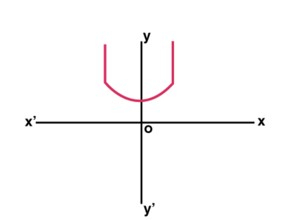# How to find the Hyperbolic Cosine of a given radian value in Golang?

In this tutorial, we will learn how to find the Hyperbolic Cosine of a given radian value in the Golang programming Language. Golang language has many packages with predefined functions that the developer can use without writing the complete logic.

To perform the mathematical operations and logic we have a math package in Golang. We will use this package only to find the Hyperbolic Cosine of a given radian value. We will also see how to import the package and also how to call a function this package consists of by writing a Golang code.

## Hyperbolic Cosine

### Definition

Hyperbolic Cosine is a function similar to trigonometry functions. The algebraic expression helps in finding the hyperbolic functions that include the exponential function(e^x). The formula for hyperbolic Cosine is written below and the value of Hyperbolic Cosine is at different angles.

### Syntax

Coshx = (e^x + e^-x) / 2


### Graph## Value of Hyperbolic Cosine at different angles

• cosh(0) = 1

• cosh(30) = 5.3432373e+12

• cosh(45) = 1.7467136e+19

• cosh(60) = 5.7100369e+25

• cosh(90) = 6.1020165e+38

### Algorithm

Step 1 - Declaring the variable to store the value of the guardian and answer of float32 type.

Step 2 - Initializing the variable of a radian.

Step 3 - Call the function of the hyperbolic Cosine and pass the radian value.

Step 4 - Printing the result.

### Example

In this example, we will write a Golang program in which we will import a math package and call the hyperbolic Cosine function.

package main
import (

// fmt package provides the function to print anything
"fmt"

// math package provides multiple functions for different

// mathematical operations
"math"
)
func main() {

// declaring the variables to store the value of radian value and answer
fmt.Println("Program to find the hyperbolic Cosine of a given radian value in the Golang programming language using a math package.")

// initializing the value of radian value

// finding Hyperbolic Cosine for the given radian value

// printing the result
}


### Output

Program to find the hyperbolic Cosine of a given radian value in the Golang programming language using a math package.
The hyperbolic Cosine value with the value of radian 4.5 is 45.014120148530026


### Algorithm

Step 1 - Declaring the variable to store the value of the guardian and answer of float32 type.

Step 2 - Initializing the variable of a radian.

Step 3 - Call the function of the hyperbolic Cosine defined by us and pass the radian value as a parameter.

Step 4 - Printing the result.

### Example

In this example, we will write a Golang program in which we will import a math package and call the hyperbolic Cosine function in a separate function and call that function main.

package main
import (

// fmt package provides the function to print anything
"fmt"

// math package provides multiple functions for different

// mathematical operations
"math"
)

// this is a function with a parameter of float64 type and a return type of float64
func HyperbolicCosine(angle float64) float64 {

// returning the Hyperbolic Cosine of the angle
return math.Cosh(angle)
}
func main() {

// declaring the variables to store the value of radian value and answer
fmt.Println("Program to find the Hyperbolic Cosine of a given radian value in the Golang programming language using a separate function in the same program.")

// initializing the value of the radian value

// finding hyperbolic Cosine for the given radian value in separate function

// printing the result
}


### Output

Program to find the Hyperbolic Cosine of a given radian value in the Golang programming language using a separate function in the same program.
The Hyperbolic Cosine value with the value of radian 4.5 is 45.014120148530026


## Conclusion

These are two ways to find the hyperbolic Cosine by using the function in the math package and passing the radian value as a parameter. The second approach will provide abstraction in the program. To learn more about Golang you can explore these tutorials.